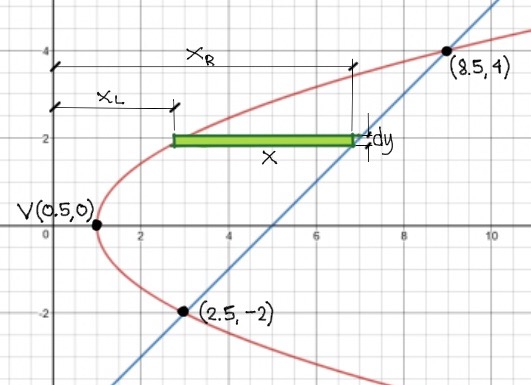# Integral calculus:Application

3 posts / 0 new
Leamir M. SakalIntegral calculus:Application

Problem: Find the area of the region bounded by the graphs as shown in the picture, given that the 4a=2.Jhun VertEquation of line:

$y - y_1 = m(x - x_1)$

$y + 2 = \dfrac{4 + 2}{8.5 - 2.5}(x - 2.5)$

$x = y + 4.5$   ←   $x_R$Equation of parabola:

$y^2 = 4a(x - h)$

$y^2 = 2(x - 0.5)$

$x = \frac{1}{2}y^2 + 1$   ←   $x_L$

$\displaystyle A = \int_{y_1}^{y_2} (x_R - x_L) \, dy$

$\displaystyle A = \int_{-2}^{4} \left[ (y + 4.5) - \left( \frac{1}{2}y^2 + 1\right) \right] \, dy$

Leamir M. SakalThanks men! Love the approach

Subscribe to MATHalino on